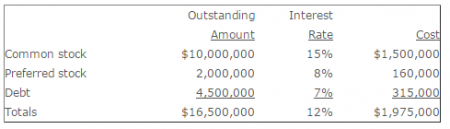# What is Weighted Average Cost of Capital?

The weighted average cost of capital (WACC) is a compilation of the aggregate financing cost of a business, where each element of its financing cost is proportionately represented. The WACC is used to discount the cash flows associated with capital budgeting proposals to determine their net present values.

Definition: Weighted Average Cost Of Capital (WACC) is the calculation of a firm’s cost of capital in which each category of capital is proportionately weighted.

The components of the cost of capital are:

• Common stock
• Preferred stock
• Debt

The following table shows a derivation of the weighted average cost of capital, where the outstanding amount of each funding source is multiplied by its related cost, to arrive at an aggregate cost percentage for all sources of revenue.When evaluating an investment proposal, the net present value calculation should only use the WACC to discount the associated cash flows if the risk profile of the investment is approximately the same as that of the firm. If the investment is more risky, then a risk premium should be added to the WACC and then used as the discount rate.

The WACC tends to decrease as a percentage when a business uses a higher proportion of debt as its source of funding, since the related interest expense is tax deductible, which reduces the cost of this form of funding. However, if too much debt is used, lenders will raise the interest rates charged, which increases the WACC. Consequently, there is a “sweet spot” where a business can take on an optimal amount of debt burden.